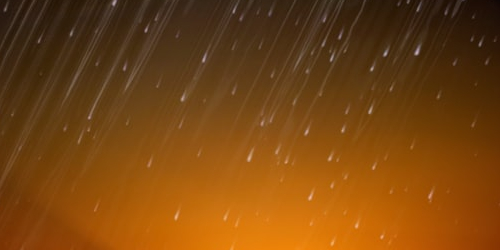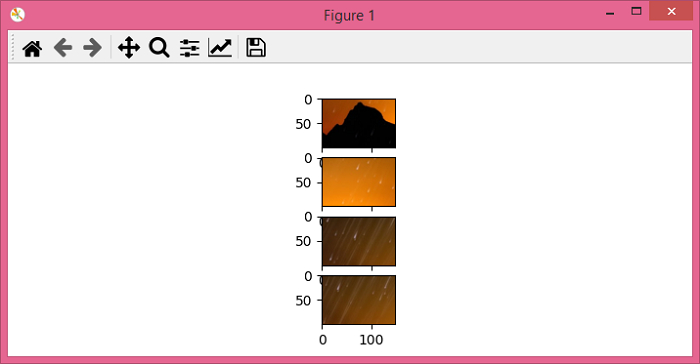# PyTorch – How to crop an image at a random location?

To crop an image at a random location, we apply RandomCrop() transformation. It's one of the many important transforms provided by the torchvision.transforms module.

The RandomCrop() transformation accepts both PIL and tensor images. A tensor image is a torch tensor with shape [C, H, W], where C is the number of channels, H is the image height and W is the image width.

If the image is neither a PIL image nor tensor image, then we first convert it to a tensor image and then apply RandomCrop().

## Syntax

torchvision.transforms.RandomCrop(size)(img)

where size is the desired crop size. size is a sequence like (h, w), where h and w are the height and width of the cropped image. If size is an int, then the cropped image will be a square image.

It returns the cropped image at a random location of given size.

## Steps

We could use the following steps to crop an image at a random location with given size −

• Import the required libraries. In all the following examples, the required Python libraries are torch, Pillow, and torchvision. Make sure you have already installed them.

import torch
import torchvision
import torchvision.transforms as T
from PIL import Image
• Read the input image. The input image is a PIL image or a torch tensor.

img = Image.open('meteor.jpg')
• Define a transform to crop the image at random location. The crop size is (200,250) for rectangular crop and 250 for square crop. Change the crop size according your need.

# transform for rectangular crop
transform = T.RandomCrop((200,250))
# transform for square crop
transform = T.RandomCrop(250)
• Apply the above-defined transform on the input image to crop the image at random location.

img = transform(img)
• Visualize the cropped image.

img.show()

## Input Image

This image is used as the input in all the following examples.## Example 1

The following Python3 program shows how to crop an input PIL image at a random location.

# import required libraries
import torch
import torchvision.transforms as T
from PIL import Image

img = Image.open('meteor.png')

# define transform to crop the image at
# random location
transform = T.RandomCrop((250,500))
img = transform(img)
img.show()

## Output

It will produce the following output −## Example 2

import torch
import torchvision.transforms as T
from PIL import Image

img = Image.open('lena.jpg')
img = transform(img)
img.show()

## Output

It will produce the following output. Notice that the padding is random.## Example 3

# import required libraries
import torch
import torchvision.transforms as T
from PIL import Image
import matplotlib.pyplot as plt

img = Image.open('meteor.png')

# define the transform with crop size
transform = T.RandomCrop((100,150))

# crop four images
imgs = [transform(img) for _ in range(4)]

# display these cropped images
fig = plt.figure(figsize=(7,3))
rows, cols = len(imgs),1
for j in range(0, len(imgs)):
plt.show()